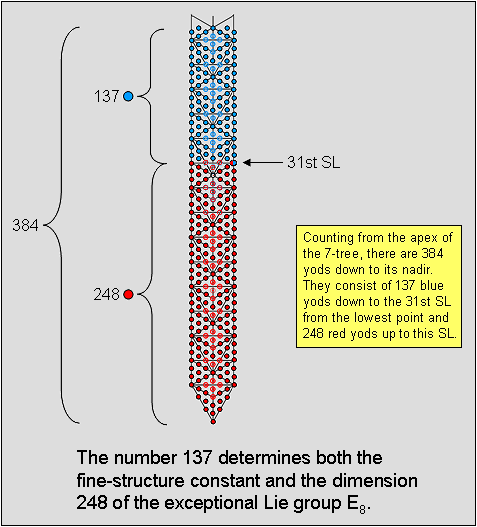<< Previous    1...   33  34    36  37  ...56    Next >>

#35 The number 137 determining the fine-structure constant also determines the dimension 248 of E8The number of yods in the n-tree when its triangles are tetractyses is given by

Y(n) = 50n + 30.*

The 7-tree maps the physical plane (physical universe). It has Y(7) = 380 yods. Two yods up to the level of its apex that belong to the eighth Tree lie outside it on each side of the central Pillar of Equilibrium. There are 384 yods up to the top of the 7-tree.

The number of yods up to Chesed of the nth Tree is given by

C(n) = 50n − 2.**

Chesed of the fifth Tree is the 31st SL, counting from the bottom of the first Tree. There are C(5) = 248 red yods up to this SL, which is denoted in the diagram opposite by a circle coloured half red and half blue for a reason to be given shortly. This shows how EL ("God"), the Godname of Chesed with number value 31, prescribes the dimension 248 of the rank-8 exceptional Lie group E8 at the heart of superstring theory. Each red yod denotes one of its 248 roots. 248 is the number value of Raziel, the Archangel of Chokmah, which is the Sephirah above Chesed on the Pillar of Mercy of the Tree of Life.

Counting from the level of the top of the 7-tree, there are (384−247=137) blue yods down to (and including) the 31st SL, which is coloured half red and half blue to indicate that it is a yod that is shared by the set of 137 blue yods and by the set of 248 red yods. But this SL is the 248th yod, counting from the base of the 7-tree. We discover the amazing fact that the number 137 determines the very dimension 248 of E8! Regarded by physicists as one of the most mysterious numbers in physics, the number 137 determines the strength of the coupling of the electric charge of the electron to the electromagnetic field because its reciprocal is the approximate value of the fine-structure constant: α = e2/ħc = 7.29752698(24)×10−3 = 1/137.0359974(44), where e is the electrical charge of the electron, ħ is the reduced Planck constant and c is the velocity of light in vacuo. The 7-tree mapping the space-time continuum (see Article 2) has the remarkable property that the 137th yod from its apex is the 248th yod from its lowest point, thereby relating through its geometry two of the most important numbers in theoretical physics. Like the number 248, the number 137 is a defining parameter of sacred geometries. Links to examples discussed in this website can be found in The holistic pattern under the heading "137".

The Pythagorean Decad relates both these numbers of deep significance to physics through the identity:

12
22  32
137 + 248 = 385 =               42  52  62
72  82  92102.

Notice that the 31st SL is the tenth SL from the bottom of the Pillar of Mercy. 31 is also the tenth prime number after 2, the first prime number. 137 is the 33rd prime number, where 33 = 1! + 2! + 3! + 4! and 10 = 1 + 2 + 3 + 4. It demonstrates the fundamental role of the tetractys in determining numbers central to superstring physics because the sum of the number of permutations of 10 objects arranged in its four rows is 33, whilst Y(33) = 1680, which is the number of circular turns that Leadbeater counted in each helical whorl of the UPA/E8×E8 heterotic superstring. Here is how the number 33 determines either arithmetically or geometrically the form of each whorl of the superstring, the symmetry group E8 governing its forces and the fine-structure constant. Its significance is far greater than what the religious, numerological and historical reasons for the association of this number with Freemasonry would lead one to expect.

* Proof: The n-tree consists of (12n+7) triangles with (6n+5) corners and (16n+9) sides. When each triangle is a tetractys, two hexagonal yods lie on each side. Number of yods in n-tree = 6n + 5 + 2×(16n+9) + 12n + 7 = 50n + 30.

** Proof: There are 18 yods outside the (n−1)-tree up to Chesed of the nth Tree of Life. The number of yods in the (n−1)-tree = Y(n−1) = 50(n−1) + 30 = 50n − 20. The number of yods up to Chesed of the nth Tree = 50n − 20 + 18 = 50n − 2.

Current recommended value.

 << Previous    1...   33  34    36  37  ...56    Next >>

Home